# Kerala Board Question Paper for SSLC Class 10th Chemistry 2015 In PDF

## KBPE 10th Standard Chemistry Exam Question Paper 2015 with Solutions – Free Download

Kerala Board SSLC Class 10 Chemistry 2015 Question Paper solutions help the students to prepare most competently and perform well in the board exams. This Class 10 exam question paper sets the foundation for all the questions that are asked in the Chemistry exam paper. Students can get the PDF formats of the solved or unsolved question paper, as their preference by clicking on the links that are mentioned in this article. For the convenience of the students, we have listed here the interactive links as well as the questions and answers on the web-page.

Every student who has mastered the entire complex topics from Chemistry will find it easier to ace the final exams. They can also easily understand the type of questions that are asked in the KBPE SSLC Class 10 Chemistry exam with the help of these question paper solutions. Students are also advised to solve the previous papers of Kerala Board Class 10 Chemistry. After solving the papers, they can refer to these solutions and gauge their performance and their preparation level for the board exams. Doing so will help them to determine where they have gone wrong and how to avoid these mistakes for the final exams. Solving these SSLC previous year question papers are the best way for the students to get an overview of the exam paper pattern and the marking schemes. Students are also able to determine the difficulty level of the exams with the help of these question paper solutions.

### Kerala Board Class 10 Chemistry 2015 Question Paper with Solutions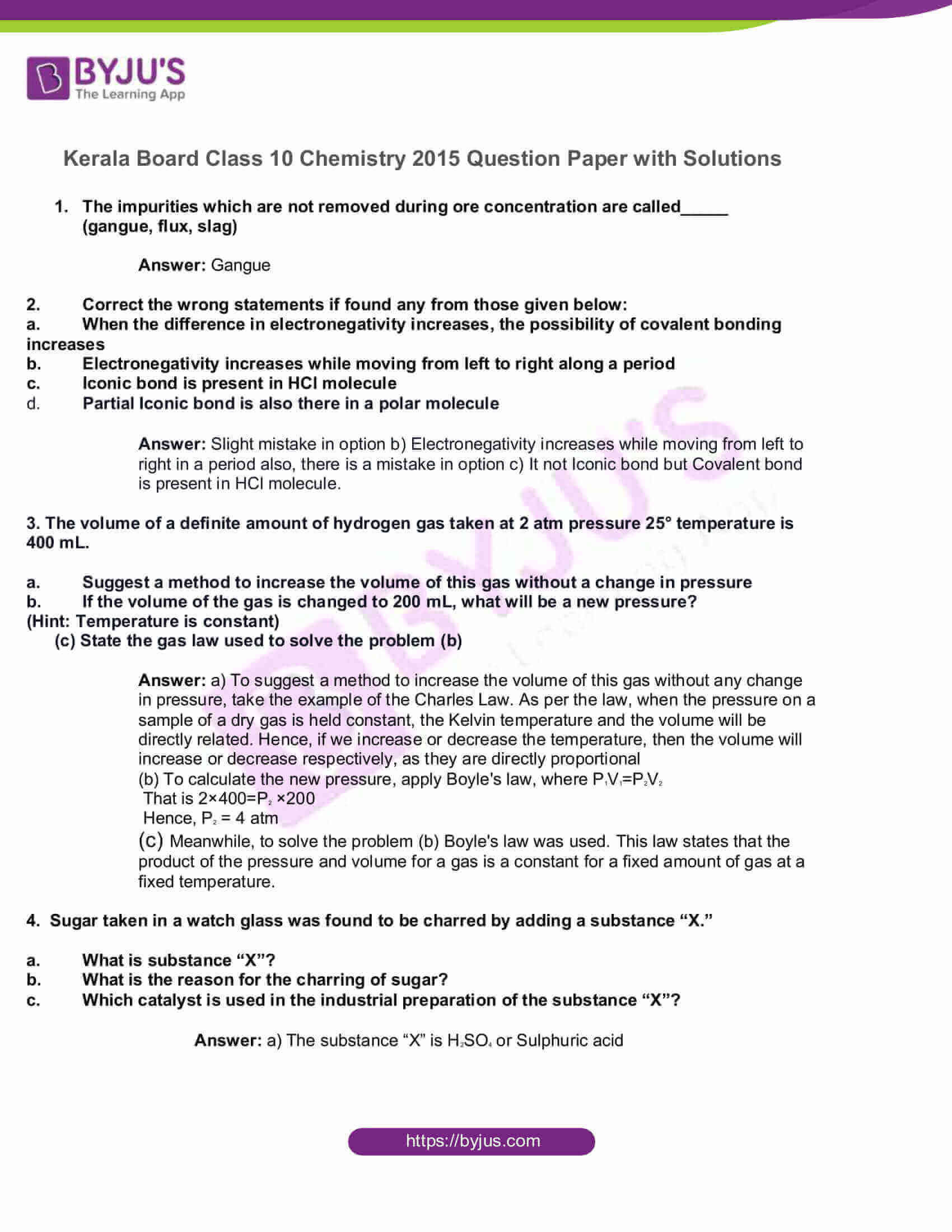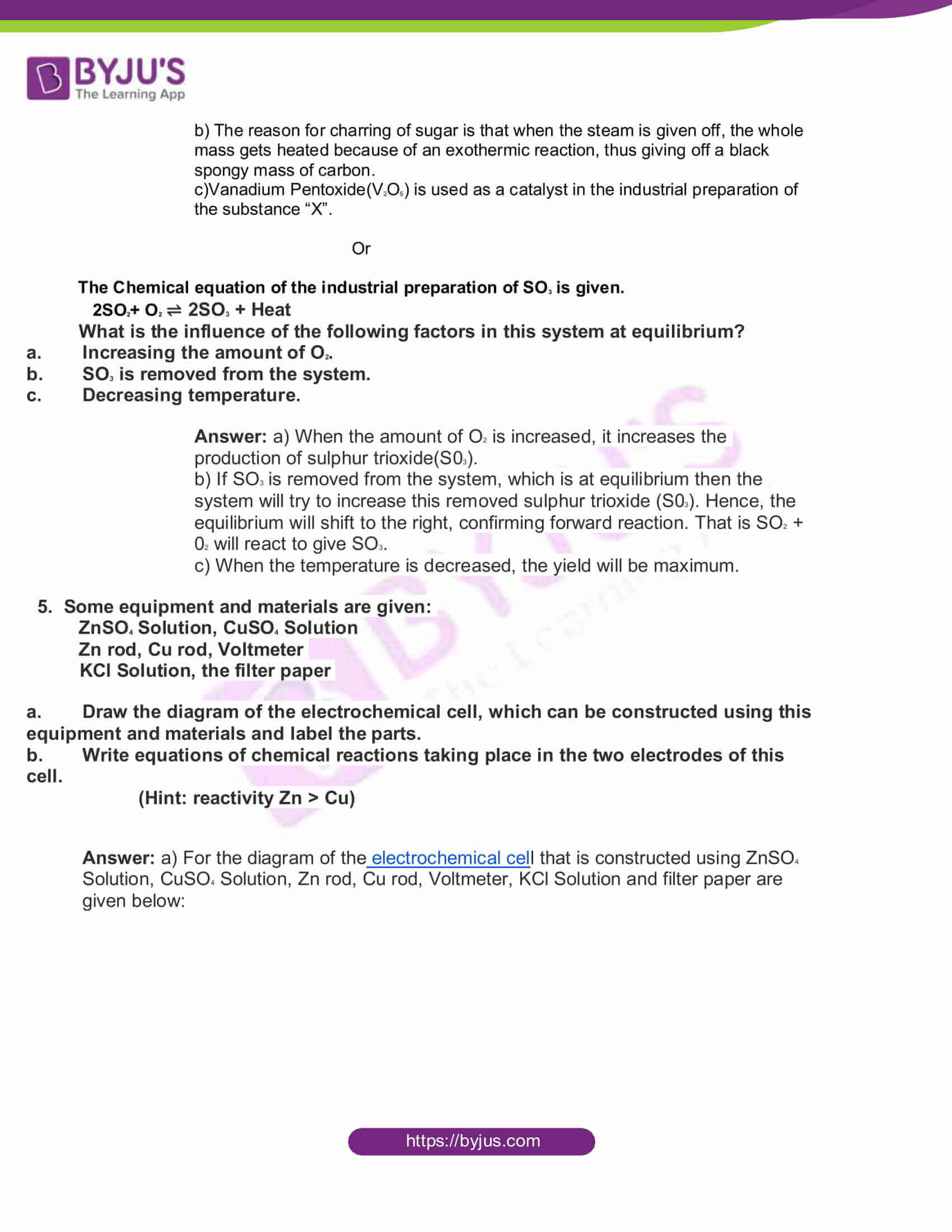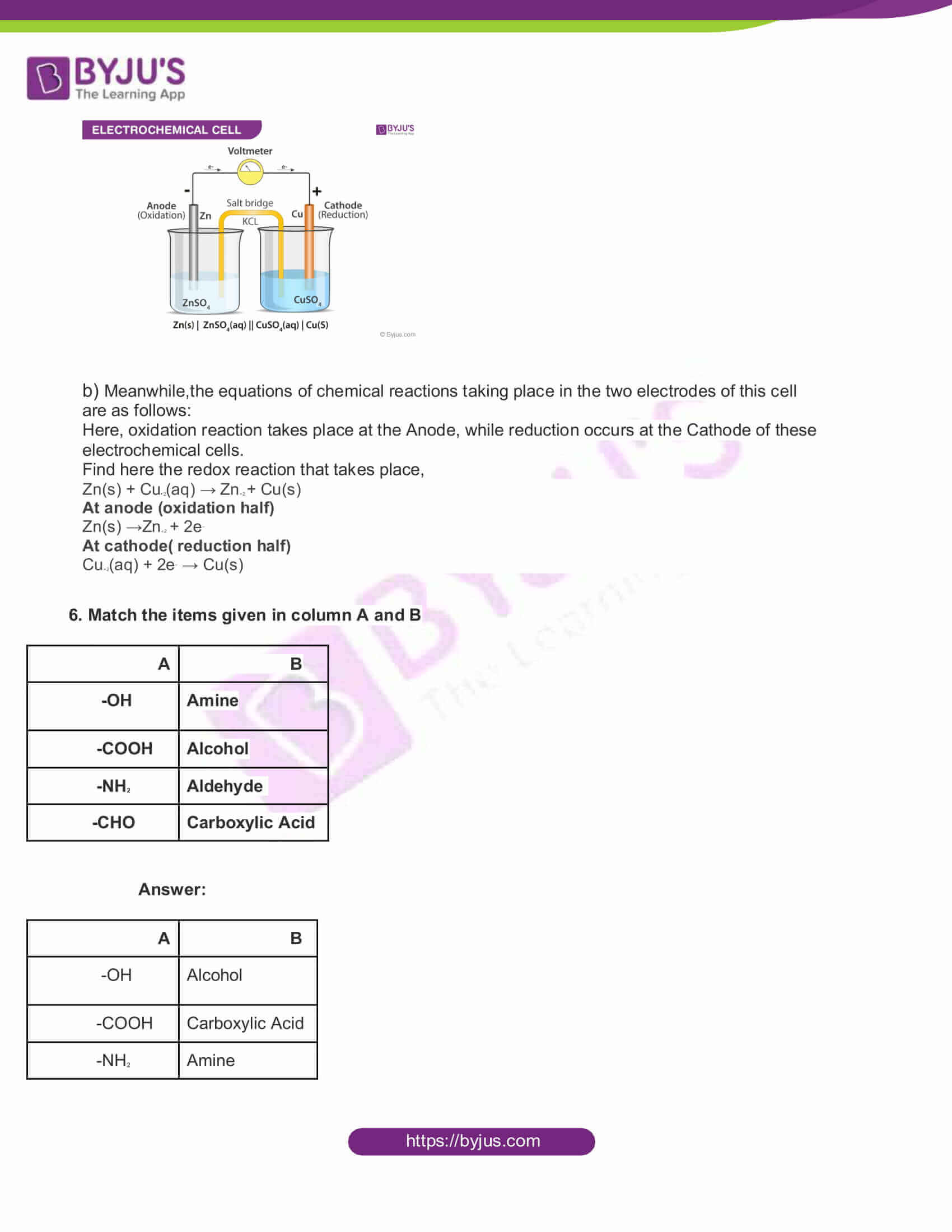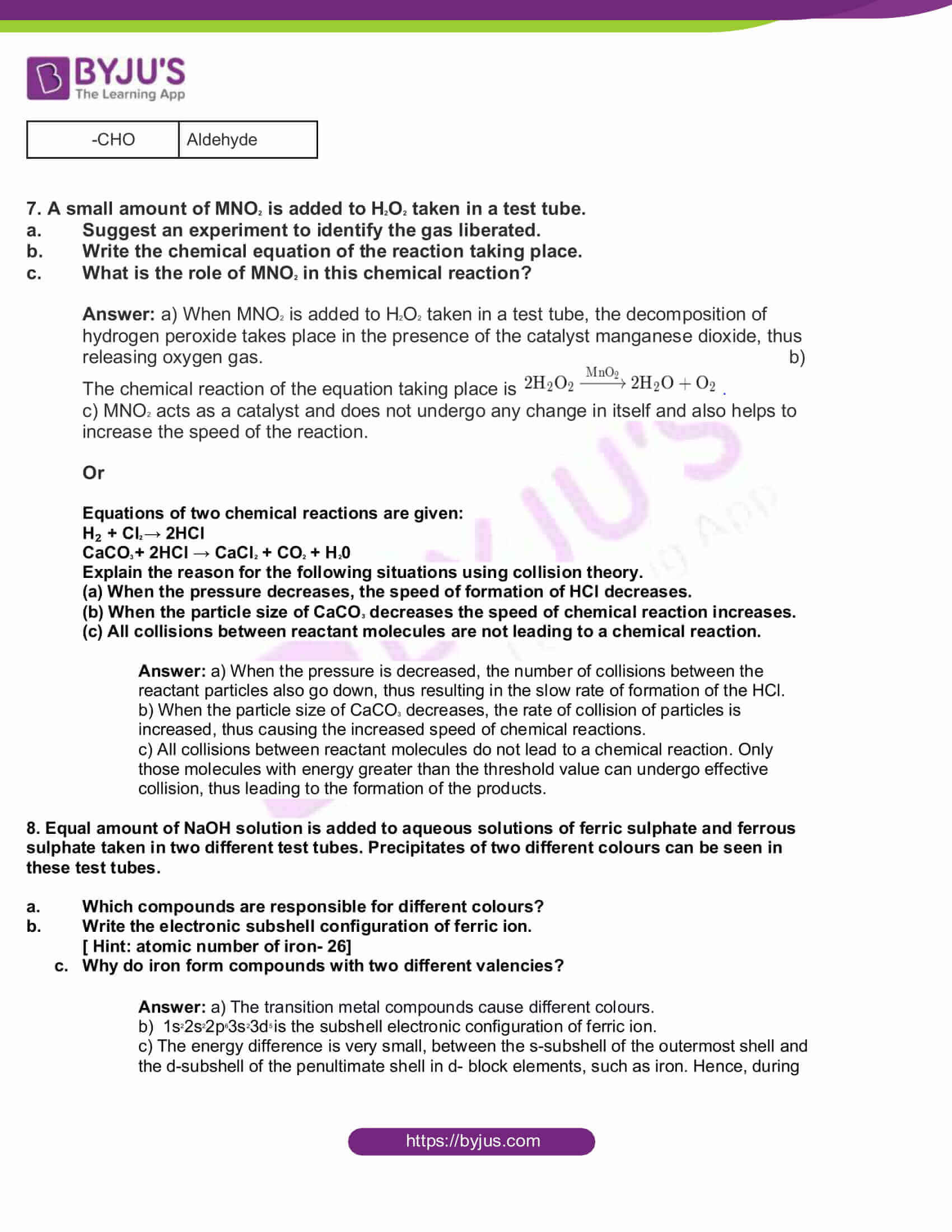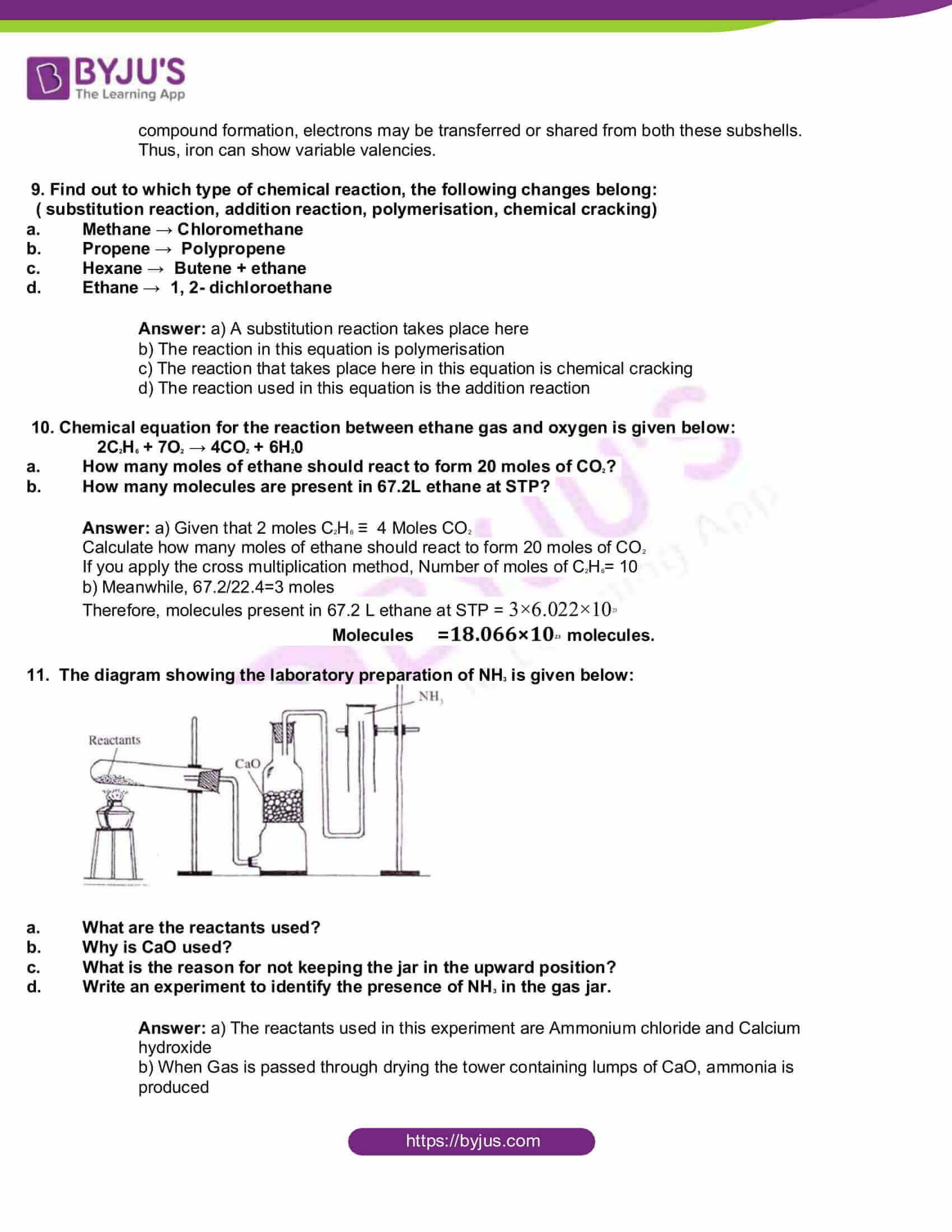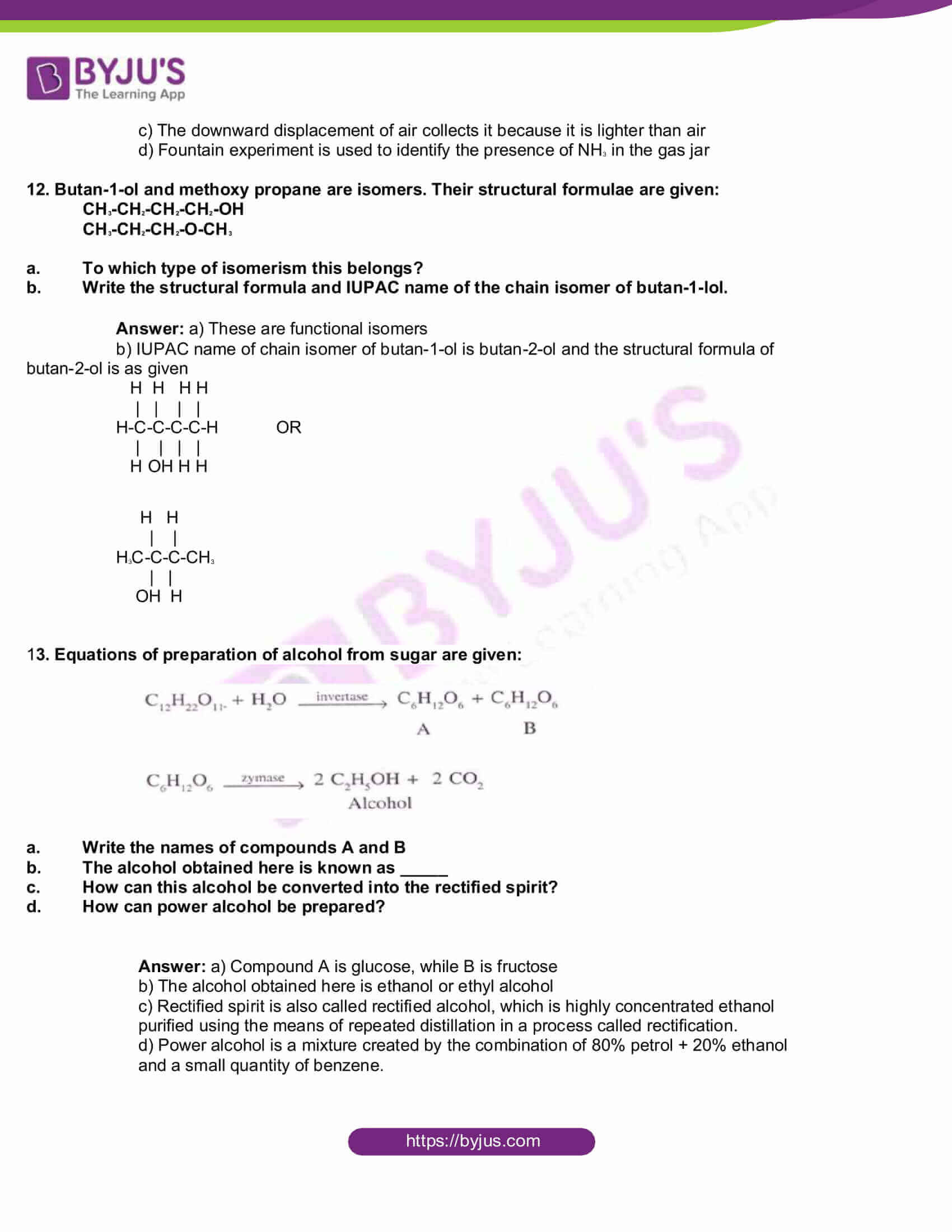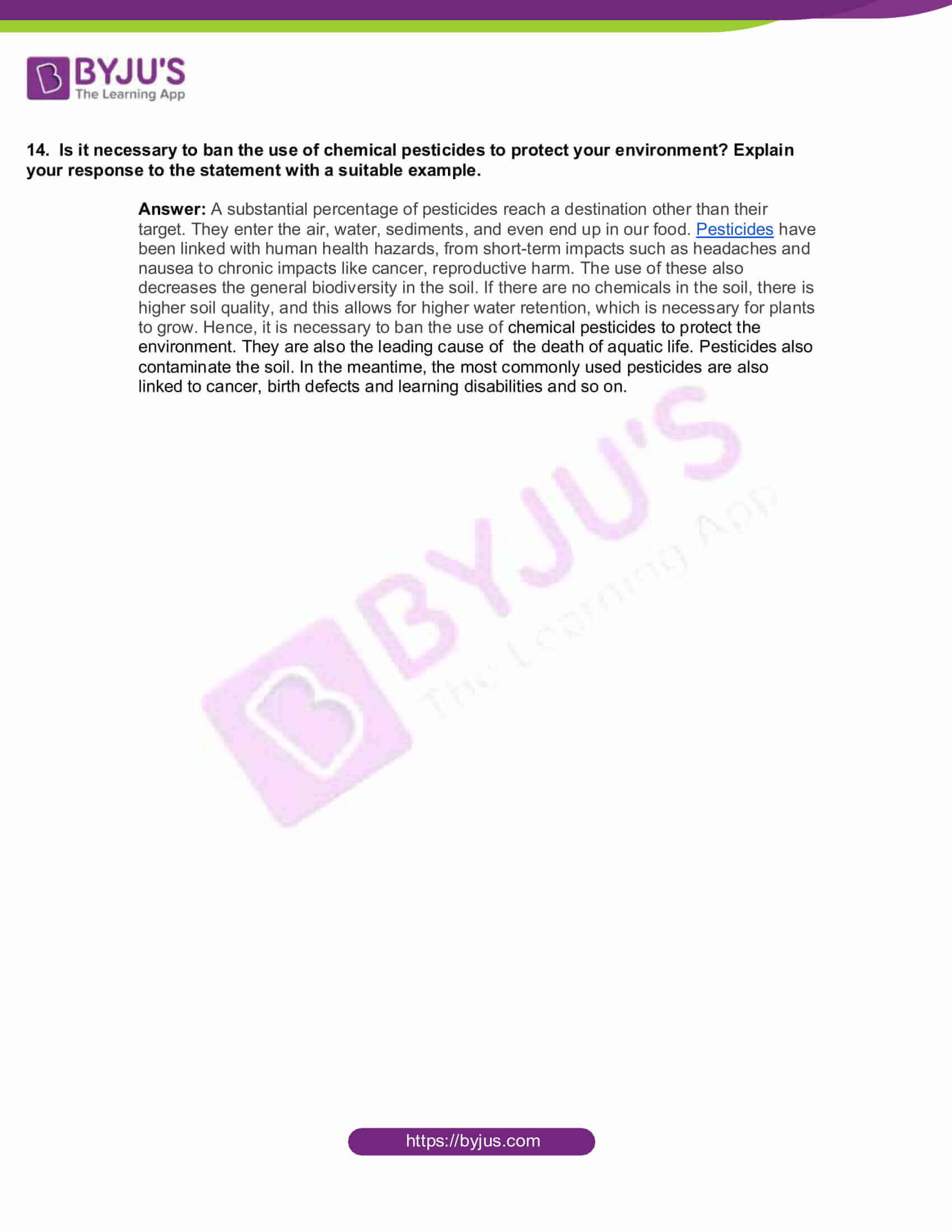1. The impurities which are not removed during ore concentration are called_____

(gangue, flux, slag)

1. Correct the wrong statements if found any from those given below:
2. When the difference in electronegativity increases, the possibility of covalent bonding increases
3. Electronegativity increases while moving from left to right along a period
4. Iconic bond is present in HCl molecule
5. Partial Iconic bond is also there in a polar molecule

Answer: Slight mistake in option b) Electronegativity increases while moving from left to right in a period also, there is a mistake in option c) It not Iconic bond but Covalent bond is present in HCl molecule.

3. The volume of a definite amount of hydrogen gas taken at 2 atm pressure 25° temperature is 400 mL.

1. Suggest a method to increase the volume of this gas without a change in pressure
2. If the volume of the gas is changed to 200 mL, what will be a new pressure?

(Hint: Temperature is constant)

(c) State the gas law used to solve the problem (b)

Answer: a) To suggest a method to increase the volume of this gas without any change in pressure, take the example of the Charles Law. As per the law, when the pressure on a sample of a dry gas is held constant, the Kelvin temperature and the volume will be directly related. Hence, if we increase or decrease the temperature, then the volume will increase or decrease respectively, as they are directly proportional

(b) To calculate the new pressure, apply Boyle’s law, where P1V1=P2V2

That is 2×400=P2 ×200

Hence, P2 = 4 atm

(c) Meanwhile, to solve the problem (b) Boyle’s law was used. This law states that the product of the pressure and volume for a gas is a constant for a fixed amount of gas at a fixed temperature.

4. Sugar taken in a watch glass was found to be charred by adding a substance “X.”

1. What is substance “X”?
2. What is the reason for the charring of sugar?
3. Which catalyst is used in the industrial preparation of the substance “X”?

Answer: a) The substance “X” is H2SO4 or Sulphuric acid

b) The reason for charring of sugar is that when the steam is given off, the whole mass gets heated because of an exothermic reaction, thus giving off a black spongy mass of carbon.

c)Vanadium Pentoxide(V2O5) is used as a catalyst in the industrial preparation of the substance “X”.

Or

The Chemical equation of the industrial preparation of SO3 is given.

2SO2+ O2 ⇌ 2SO3 + Heat

What is the influence of the following factors in this system at equilibrium?

1. Increasing the amount of O2.
2. SO3 is removed from the system.
3. Decreasing temperature.

Answer: a) When the amount of O2 is increased, it increases the production of sulphur trioxide(S03).

b) If SO3 is removed from the system, which is at equilibrium then the system will try to increase this removed sulphur trioxide (S03). Hence, the equilibrium will shift to the right, confirming forward reaction. That is SO2 + 02 will react to give SO3.

c) When the temperature is decreased, the yield will be maximum.

5. Some equipment and materials are given:

ZnSO4 Solution, CuSO4 Solution

Zn rod, Cu rod, Voltmeter

KCl Solution, the filter paper

1. Draw the diagram of the electrochemical cell, which can be constructed using this equipment and materials and label the parts.
2. Write equations of chemical reactions taking place in the two electrodes of this cell.

(Hint: reactivity Zn > Cu)

Answer: a) For the diagram of the electrochemical cell that is constructed using ZnSO4 Solution, CuSO4 Solution, Zn rod, Cu rod, Voltmeter, KCl Solution and filter paper are given below: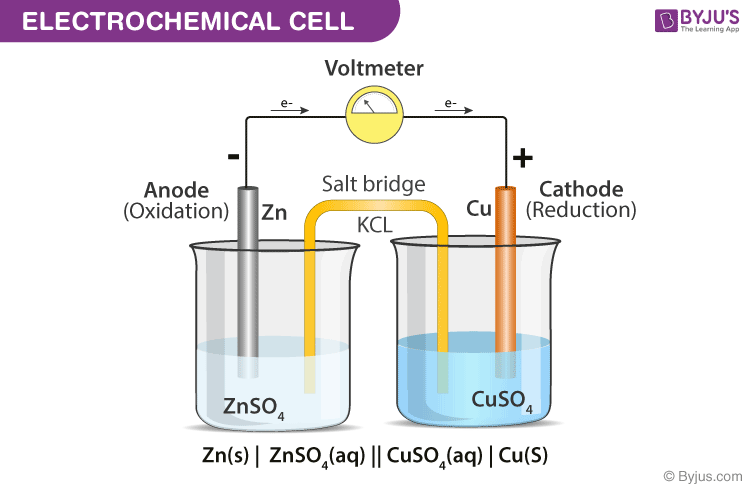b) Meanwhile,the equations of chemical reactions taking place in the two electrodes of this cell are as follows:

Here, oxidation reaction takes place at the Anode, while reduction occurs at the Cathode of these electrochemical cells.

Find here the redox reaction that takes place,

Zn(s) + Cu+2(aq) → Zn+2 + Cu(s)

At anode (oxidation half)

Zn(s) →Zn+2 + 2e

At cathode( reduction half)

Cu+2(aq) + 2e → Cu(s)

6. Match the items given in column A and B

 A B -OH Amine -COOH Alcohol -NH2 Aldehyde -CHO Carboxylic Acid

 A B -OH Alcohol -COOH Carboxylic Acid -NH2 Amine -CHO Aldehyde

7. A small amount of MNO2 is added to H2O2 taken in a test tube.

1. Suggest an experiment to identify the gas liberated.
2. Write the chemical equation of the reaction taking place.
3. What is the role of MNO2 in this chemical reaction?

Answer: a) When MNO2 is added to H2O2 taken in a test tube, the decomposition of hydrogen peroxide takes place in the presence of the catalyst manganese dioxide, thus releasing oxygen gas. b) The chemical reaction of the equation taking place is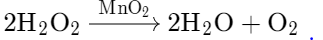c) MNO2 acts as a catalyst and does not undergo any change in itself and also helps to increase the speed of the reaction.

Or

Equations of two chemical reactions are given:

H₂ + Cl2 → 2HCl

CaCO3 + 2HCl → CaCl2 + CO2 + H20

Explain the reason for the following situations using collision theory.

(a) When the pressure decreases, the speed of formation of HCl decreases.

(b) When the particle size of CaCO3 decreases the speed of chemical reaction increases.

(c) All collisions between reactant molecules are not leading to a chemical reaction.

Answer: a) When the pressure is decreased, the number of collisions between the reactant particles also go down, thus resulting in the slow rate of formation of the HCl.

b) When the particle size of CaCO3 decreases, the rate of collision of particles is increased, thus causing the increased speed of chemical reactions.

c) All collisions between reactant molecules do not lead to a chemical reaction. Only those molecules with energy greater than the threshold value can undergo effective collision, thus leading to the formation of the products.

8. Equal amount of NaOH solution is added to aqueous solutions of ferric sulphate and ferrous sulphate taken in two different test tubes. Precipitates of two different colours can be seen in these test tubes.

1. Which compounds are responsible for different colours?
2. Write the electronic subshell configuration of ferric ion.

[ Hint: atomic number of iron- 26]

1. Why do iron form compounds with two different valencies?

Answer: a) The transition metal compounds cause different colours.

b) 1s22s22p63s23d5 is the subshell electronic configuration of ferric ion.

c) The energy difference is very small, between the s-subshell of the outermost shell and the d-subshell of the penultimate shell in d- block elements, such as iron. Hence, during compound formation, electrons may be transferred or shared from both these subshells. Thus, iron can show variable valencies.

9. Find out to which type of chemical reaction, the following changes belong:

( substitution reaction, addition reaction, polymerisation, chemical cracking)

1. Methane → Chloromethane
2. Propene → Polypropene
3. Hexane → Butene + ethane
4. Ethane → 1, 2- dichloroethane

Answer: a) A substitution reaction takes place here

b) The reaction in this equation is polymerisation

c) The reaction that takes place here in this equation is chemical cracking

d) The reaction used in this equation is the addition reaction

10. Chemical equation for the reaction between ethane gas and oxygen is given below:

2C2H6 + 7O2 → 4CO2 + 6H20

1. How many moles of ethane should react to form 20 moles of CO2?
2. How many molecules are present in 67.2L ethane at STP?

Answer: a) Given that 2 moles C2H6 ≡ 4 Moles CO2

Calculate how many moles of ethane should react to form 20 moles of CO2

If you apply the cross multiplication method, Number of moles of C2H6= 10

b) Meanwhile, 67.2/22.4=3 moles

Therefore, molecules present in 67.2 L ethane at STP = 3×6.022×1023

## Molecules =18.066×1023 molecules.

11. The diagram showing the laboratory preparation of NH3 is given below: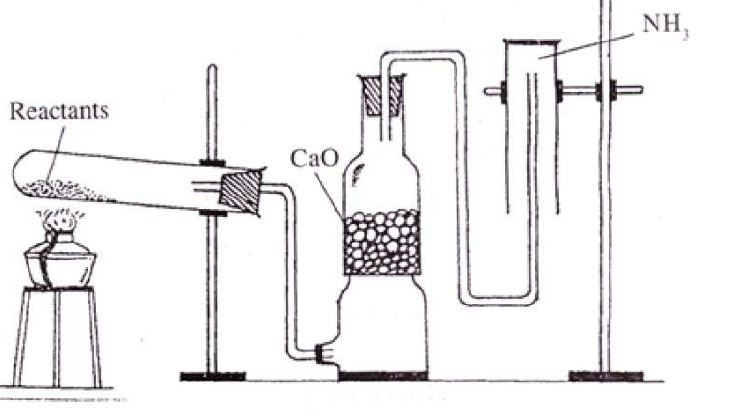1. What are the reactants used?
2. Why is CaO used?
3. What is the reason for not keeping the jar in the upward position?
4. Write an experiment to identify the presence of NH3 in the gas jar.

Answer: a) The reactants used in this experiment are Ammonium chloride and Calcium hydroxide

b) When Gas is passed through drying the tower containing lumps of CaO, ammonia is produced

c) The downward displacement of air collects it because it is lighter than air

d) Fountain experiment is used to identify the presence of NH3 in the gas jar

12. Butan-1-ol and methoxy propane are isomers. Their structural formulae are given:

CH3-CH2-CH2-CH2-OH

CH3-CH2-CH2-O-CH3

1. To which type of isomerism this belongs?
2. Write the structural formula and IUPAC name of the chain isomer of butan-1-lol.

Answer: a) These are functional isomers

b) IUPAC name of chain isomer of butan-1-ol is butan-2-ol and the structural formula of butan-2-ol is as given

H H H H

| | | |

H-C-C-C-C-H OR

| | | |

H OH H H

H H

| |

H3C-C-C-CH3

| |

OH H

13. Equations of preparation of alcohol from sugar are given: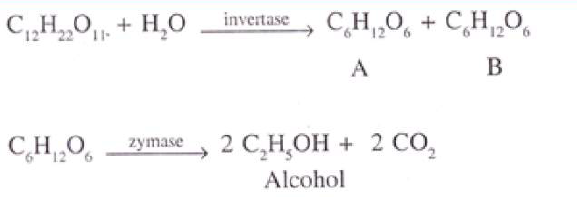1. Write the names of compounds A and B
2. The alcohol obtained here is known as _____
3. How can this alcohol be converted into the rectified spirit?
4. How can power alcohol be prepared?

Answer: a) Compound A is glucose, while B is fructose

b) The alcohol obtained here is ethanol or ethyl alcohol

c) Rectified spirit is also called rectified alcohol, which is highly concentrated ethanol purified using the means of repeated distillation in a process called rectification.

d) Power alcohol is a mixture created by the combination of 80% petrol + 20% ethanol and a small quantity of benzene.

14. Is it necessary to ban the use of chemical pesticides to protect your environment? Explain your response to the statement with a suitable example.

Answer: A substantial percentage of pesticides reach a destination other than their target. They enter the air, water, sediments, and even end up in our food. Pesticides have been linked with human health hazards, from short-term impacts such as headaches and nausea to chronic impacts like cancer, reproductive harm. The use of these also decreases the general biodiversity in the soil. If there are no chemicals in the soil, there is higher soil quality, and this allows for higher water retention, which is necessary for plants to grow. Hence, it is necessary to ban the use of chemical pesticides to protect the environment. They are also the leading cause of the death of aquatic life. Pesticides also contaminate the soil. In the meantime, the most commonly used pesticides are also linked to cancer, birth defects and learning disabilities and so on.